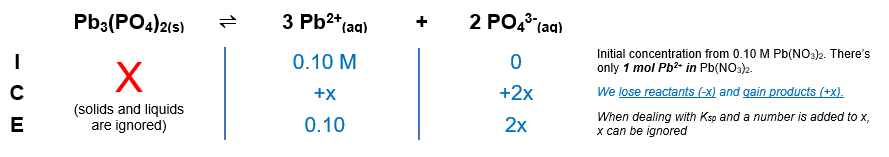# Problem: Calculate the solubility of solid Pb3(PO4)2 (Ksp = 1 X 10-54) in a 0.10-M Pb(NO3)2 solution.

###### FREE Expert Solution

Pb3(PO4)2(s)  3 Pb2+(aq) + 2 PO43-(aq)

Pb(NO3)2(aq) Pb2+(aq) + 2 NO3-(aq)${\mathbf{K}}_{\mathbf{sp}}\mathbf{=}\frac{\mathbf{products}}{\overline{)\mathbf{reactants}}}\phantom{\rule{0ex}{0ex}}{\mathbf{K}}_{\mathbf{sp}}\mathbf{=}{\mathbf{\left[}{\mathbf{Pb}}^{\mathbf{2}\mathbf{+}}\mathbf{\right]}}^{\mathbf{3}}{\mathbf{\left[}{{\mathbf{PO}}_{\mathbf{4}}}^{\mathbf{3}\mathbf{-}}\mathbf{\right]}}^{\mathbf{2}}$

97% (97 ratings)###### Problem Details

Calculate the solubility of solid Pb3(PO4)2 (Ksp = 1 X 10-54) in a 0.10-M Pb(NO3)2 solution.